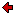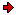Journal of Technical
Physics

A QUARTERLY JOURNALPrevious issue vol. 44, no. 1 (2003) vol. 44, no. 2 (2003)Next issuevol. 44, no. 3 (2003)

### Contents of issue 2, vol. 44

1. S. Piekarski: On the modified Fick law and its potential applications
2. K. Pawluk: Integrals of Lipschitz-Hankel type in analysis of magnetostatic fields
3. T. J. Hoffmann D. Wróbel: Extended phenomenological electrodynamics of dye-solvent mixture
4. V. Henč-Bartolić, E. Kovačević, H.-J. Kunze, M. Stubičar: Laser-produced copper plasmas
5. B. Atamaniuk K. Żuchowski: Charge fluctuation of dust grains and its impact on dusty wave propagation
6. J. Avsec: Calculation of equilibrium and nonequilibrium thermophysical properties by means of statistical mechanics
7. A. Ciarkowski: Dependence of the Brillouin precursor form on the initial signal rise-time
8. A. J. Turski, B. Atamaniuk, E. Turska: Fractional derivative analysis of Helmholtz and paraxial-wave equations
9. A. Chanda, J. Das: Measures of complexity in wind direction
10. R. Brojewski, J. Jasiński: Influence of the method of dry convection in the atmosphere modeling on the dynamic properties of the model

S. Piekarski: On the modified Fick law and its potential applications
It can be observed that the standard Einstein-Smoluchowski equation for isothermal processes can be written in the two equivalent forms which, however, become distinct when the temperature becomes spatially varying. Therefore, we have two different equations which could potentially describe the flux of diffusing particles subjected to the potential external forces and variable temperature. The first is the standard Einstein-Smoluchowski equation, while the second is its modification containing an additional term proportional to the temperature gradient. In order to fix the terminology, the above mentioned modification of standard Einstein-Smoluchowski equation shall be called "the modified Fick law". In this paper, we shall discuss the origin of this equation and we shall try to make a preliminary discussion of its solutions and potential applications to thermodiffusion. We propose two models: one exploiting Onsager's thermodynamics and the second one independent from it. Elementary solutions of both models are identical in the limit of small concentrations.

K. Pawluk: Integrals of Lipschitz-Hankel type in analysis of magnetostatic fields
A boundary-integral model of the static magnetic field due to cylindrical permanent magnets that is put in free space is considered. Magnetic scalar potential quantities created by a virtual quantity "surface magnetic charge density" is expressed by means of Lipschitz-Hankel integrals that for the considered case are reducible (by the way of hypergeometric series) to some algebraic expressions, in which elliptic integrals of various kinds occur. This approach seems to be more effective than that can be reached by the use of a typical professional software for the field problems in which numerical integration, being not quite conform to the considered case, is common. The magnet subjected to the analysis has to be virtually subdivided in some number of elementary pieces, inside of which the uniform distribution of the inherent magnetization is supposed.

T. J. Hoffmann D. Wróbel: Extended phenomenological electrodynamics of dye-solvent mixture
In the paper, the description of dynamics of the electromagnetic fields in dye-solvent mixture in the concept of extended phenomenological electrodynamics is presented. For the description of the structure of material, a phenomenological model of the multi-component body is used. Such a model is based on the following assumptions:
1) a body consists of many homogeneously (ideally mixed) different components and
2) all components are located in each space point occupied by the body.
It seems that the dye-solvent mixture fulfils the above phenomenological supposition for the multi-component body. Dynamics of the electromagnetic field has been expressed in the Lagrange--Hamiltonian formalism, and can be used in investigation of the local electromagnetic field in dye-solvent mixture.

V. Henč-Bartolić, E. Kovačević, H.-J. Kunze, M. Stubičar: Laser-produced copper plasmas
The Cu plasmas were produced by a ruby laser (5J, 694.3nm, 18ns, 140GWcm-2). Time-resolved spectra of the plasmas were observed in the region of 8-24 nm. The electron temperature was estimated to be about 40eV. A small depth of the crater (3μm) and the surrounding ripples were observed at the Cu target surface. The trace of deposited plasma was explained as the Rayleigh-Taylor instability. Plasma was transparent to the laser beam.

B. Atamaniuk K. Żuchowski: Charge fluctuation of dust grains and its impact on dusty wave propagation
In this paper we consider the influence of dust charge fluctuations on damping of the dust-ion-acoustic waves. Fluid approximation of longitudinal electrostatic waves in unmagnetized plasmas is considered. We show that for a weak acoustic wave the attenuation depends on a phenomenological charging coefficient.

J. Avsec : Calculation of equilibrium and nonequilibrium thermophysical properties by means of statistical mechanics
The paper features the mathematical model of computing equilibrium and nonequilibrium thermophysical properties of state in the liquid and gas domain for pure refrigerants and mixtures with the help of classical thermodynamics and statistical thermodynamics. The paper features all important contributions (translation, rotation, internal rotation, vibration, intermolecular potential energy and influence of electron and nuclei excitation). To calculate the thermodynamic properties of real fluid, the models on the basis of Lennard-Jones intermolecular potential were applied. To calculate the thermodynamic properties of real fluid by means of classical thermodynamics, we used the Tillner-Roth-Watanabe-Wagner (TRWW) equation on the base of Helmholtz type. We have developed a mathematical model for the calculation of all equilibrium and nonequilibrium thermodynamic functions of state for pure refrigerants. The analytical results obtained by statistical thermodynamics are compared with the TRWW model and show a relatively good agreement.

A. Ciarkowski: Dependence of the Brillouin precursor form on the initial signal rise-time
Propagation of a Brillouin precursor in a Lorentz dispersive medium is considered. The precursor is excited by a sine-modulated initial signal, with its envelope described by a hyperbolic tangent function. The purpose of the paper is to show how the rate of growth of the signal affects the form of the Brillouin precursor. Uniform asymptotic approach, pertinent to coalescing saddle points, is applied in the analysis. The results are illustrated with numerical examples. Key words: Lorentz medium, dispersive propagation, Brillouin precursor, uniform asymptotic expansions.

A. J. Turski, B. Atamaniuk, E. Turska: Fractional derivative analysis of Helmholtz and paraxial-wave equations
Fundamental rules and definitions of Fractional Calculus are outlined. Factorizing 1-D and 2-D Helmholtz equations, four semi-differential eigenfunctions are determined. The functions exhibit incident and reflected plane waves as well as diffracted incident and reflected waves on the half-plane edge. They allow to construct the Sommerfeld half-plane diffraction solutions. Parabolic-Wave Equation (PWE, Leontovich-Fock) for paraxial propagation is factorized and differential fractional solutions of Fresnel-integral type are determined. We arrived at two solutions, which are the mothers of known and new solutions.

A. Chanda, J. Das: Measures of complexity in wind direction
In the present paper an attempt has been made to utilise two different forms of complexity measures in directional change of wind. Two types of complexity measures are average mutual information and multifractal dimensions. Four sets of data have been studied. For each set, all the three measures have been calculated. From the results, a comparative study of the properties of the two measures of complexity vis-a-vis understanding the nature of complexity has been done.

R. Brojewski, J. Jasiński: Influence of the method of dry convection in the atmosphere modeling on the dynamic properties of the model
This paper presents the problem of modeling dry convection in the atmosphere based on scaling of the movement equations resulting from the assumption that convection streams are mainly generated by the Archimedes draught force. This approach leads to description of the atmosphere movement different than in the Boussinesq approximation. The simplest case of Galerkin type equations in 3D phase space was considered. The obtained equations have different dynamics than the equations of the classical Lorenz model of dry convection. Lorenz model dynamics is controlled by the configuration of 2 non-dimensional numbers, while the dynamics of the proposed model is controlled by 3 numbers. It is presented in the language of symbolic dynamics, illustrated with numerous examples - indicating its different character than in the classical Lorenz model, among others: different values of Rayleigh number for which the systems loose structural stability.

Reprints of the full papers may be obtained from their authors. Contact Editorial Office in case you need the address of the respective author.
 Home Editors Scope Abstracts For authors Subscription